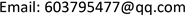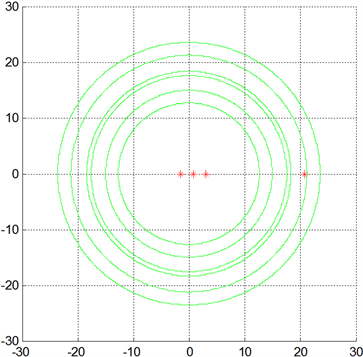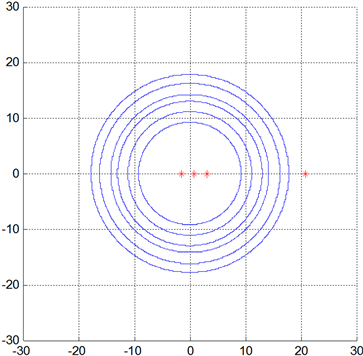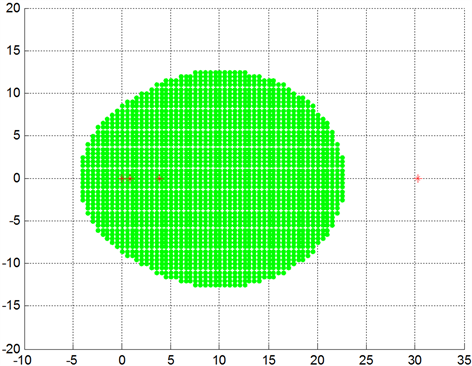﻿ 正定矩阵的判定方法和新的Brauer卵形 Determination Method of Positive Definite Matrix and New Brauer Oval

Pure Mathematics
Vol.08 No.01(2018), Article ID:23351,8 pages
10.12677/PM.2018.81003

Determination Method of Positive Definite Matrix and New Brauer Oval

Qiaojuan Zheng

School of Mathematics and Statistics, Yunnan University, Kunming YunnanReceived: Dec. 18th, 2017; accepted: Jan. 1st, 2018; published: Jan. 8th, 2018ABSTRACT

The eigenvalue inclusion region in [Qiaojuan Zheng, Yaotang Li. p-Norm DSDD Matrices and New Eigenvalue Localization Region, Applied Mathematical Progress, 2017, 6(3): 367-375.] is used to obtain a method for determining the positive definite property of the real symmetric matrix. In addition, a new normal matrix Brauer oval eigenvalue inclusion region is given, so that each oval contains at least one eigenvalue of the matrix.

Keywords:p-norm DSDD Matrix, Real Symmetric Matrix, Positive Definite Property, Eigenvalue Contain Region1. 引言

2. 预备知识

${‖x‖}_{q}={\left(\sum _{i\in N}{|{x}_{i}|}^{q}\right)}^{\frac{1}{q}}$${r}_{i}^{p}\left(A\right)={\left(\sum _{j\in N\\left\{i\right\}}{|{a}_{ij}|}^{p}\right)}^{\frac{1}{p}}$$i\in N$

${x}^{\text{T}}Ax>0\text{\hspace{0.17em}}\left({x}^{\text{T}}Ax\ge 0\right)$ (2.1)

$|{a}_{ii}|\cdot |{a}_{jj}|\ge {r}_{i}\left(A\right){r}_{j}\left(A\right)$$i,j\in N$ ，且 $i\ne j$ (2.2)

$|{a}_{ii}|\cdot |{a}_{jj}|>{r}_{i}\left(A\right){r}_{j}\left(A\right)$$i,j\in N$ ，且 $i\ne j$

$\sigma \left(A\right)\subseteq K\left(A\right)=\underset{\begin{array}{l}i,j\in N\\ i\ne j\end{array}}{\cup }{K}_{i,j}\left(A\right)$ (2.3)

${K}_{i,j}\left(A\right)=\left\{z\in C:|z-{a}_{ii}|\cdot |z-{a}_{jj}|\le {r}_{i}\left(A\right){r}_{j}\left(A\right)\right\}$ ,

${x}_{i}{x}_{j}|{a}_{ii}||{a}_{jj}|>{r}_{i}^{p}\left(A\right){r}_{j}^{p}\left(A\right),\text{\hspace{0.17em}}i,j\in N;\text{\hspace{0.17em}}i\ne j$ (2.4)

$\sum _{\begin{array}{l}i,j\in N\\ i\ne j\end{array}}{\left(\frac{{r}_{i}^{p}\left(A\right){r}_{j}^{p}\left(A\right)}{|{a}_{ii}||{a}_{jj}|}\right)}^{\frac{p}{p-1}}<1$(2.5)

$\sigma \left(A\right)\subseteq {\Phi }^{p,x}\left(A\right):=\underset{{‖x‖}_{q}\le 1}{\cap }\underset{i,j\in N}{\cup }{\Phi }_{i,j}^{p,x}\left(A\right)$(2.6)

$\sigma \left(A\right)\subseteq {\Phi }^{p}\left( A \right)$

${\Phi }^{p}\left(A\right):=\left\{z\in C:\sum _{\begin{array}{l}i,j\in N\\ i\ne j\end{array}}{\left(\frac{{r}_{i}^{p}\left(A\right){r}_{j}^{p}\left(A\right)}{|z-{a}_{ii}|\cdot |z-{a}_{jj}|}\right)}^{\frac{p}{p-1}}\ge 1\right\}$ (2.7)

$\sum _{\begin{array}{l}i,j\in N\\ i\ne j\end{array}}{\left(\frac{{r}_{i}^{p}\left(A\right){r}_{j}^{p}\left(A\right)}{|z-{a}_{ii}|\cdot |z-{a}_{jj}|}\right)}^{\frac{p}{p-1}}<1$(2.8)

$B=\lambda I-A=\left({b}_{ij}\right)$ ，由 $\lambda \in \sigma \left(A\right)$ 知， $0\in \sigma \left(B\right)$ ，故 $B$ 为奇异矩阵。由 $B=\lambda I-A=\left({b}_{ij}\right)$

${r}_{i}^{p}\left(B\right)={r}_{i}^{p}\left(A\right)$$|{b}_{ii}|=|\lambda -{a}_{ii}|$$i\in N$

${r}_{j}^{p}\left(B\right)={r}_{j}^{p}\left(A\right)$$|{b}_{jj}|=|\lambda -{a}_{jj}|$$j\in N$

$\sum _{\begin{array}{l}i,j\in N\\ i\ne j\end{array}}{\left(\frac{{r}_{i}^{p}\left(B\right){r}_{j}^{p}\left(B\right)}{|{b}_{ii}|\cdot |{b}_{jj}|}\right)}^{\frac{p}{p-1}}<1$

3. 实对称矩阵正定性的判定

$\lambda \in {\Phi }_{{i}_{0},{j}_{0}}^{p,x}\left( A \right)$

${x}_{{i}_{0}}{x}_{{j}_{0}}|\lambda -{a}_{{i}_{0}{i}_{0}}||\lambda -{a}_{{j}_{0}{j}_{0}}|\le {r}_{{i}_{0}}^{p}\left(A\right){r}_{{j}_{0}}^{p}\left(A\right)$ (3.1)

${x}_{{i}_{0}}{x}_{{j}_{0}}|\lambda -{a}_{{i}_{0}{i}_{0}}||\lambda -{a}_{{j}_{0}{j}_{0}}|\ge {x}_{{i}_{0}}{x}_{{j}_{0}}{a}_{{i}_{0}{i}_{0}}{a}_{{j}_{0}{j}_{0}}>{r}_{{i}_{0}}^{p}\left(A\right){r}_{{j}_{0}}^{p}\left( A \right)$

$\sum _{\begin{array}{l}i,j\in N\\ i\ne j\end{array}}{\left(\frac{{r}_{i}^{p}\left(A\right){r}_{j}^{p}\left(A\right)}{|{a}_{ii}||{a}_{jj}|}\right)}^{\frac{p}{p-1}}\le 1$ (3.2)

$A$ 是正半定的。

$\underset{\begin{array}{l}i,j\in N,\\ i\ne j\end{array}}{\mathrm{max}}\frac{{r}_{i}\left(A\right){r}_{j}\left(A\right)}{|{a}_{ii}||{a}_{jj}|}\le 1$

$\sum _{\begin{array}{l}i,j\in N\\ i\ne j\end{array}}{\left(\frac{{r}_{i}^{p}\left(A\right){r}_{j}^{p}\left(A\right)}{|\lambda -{a}_{ii}|\cdot |\lambda -{a}_{jj}|}\right)}^{\frac{p}{p-1}}\ge 1$

$\sum _{\begin{array}{l}i,j\in N\\ i\ne j\end{array}}{\left(\frac{{r}_{i}^{p}\left(A\right){r}_{j}^{p}\left(A\right)}{|{a}_{ii}|\cdot |{a}_{jj}|}\right)}^{\frac{p}{p-1}}>\sum _{\begin{array}{l}i,j\in N\\ i\ne j\end{array}}{\left(\frac{{r}_{i}^{p}\left(A\right){r}_{j}^{p}\left(A\right)}{|\lambda -{a}_{ii}|\cdot |\lambda -{a}_{jj}|}\right)}^{\frac{p}{p-1}}\ge 1$

4. 新的Brauer卵形

$A{A}^{\ast }={A}^{\ast }A$

${E}_{i}=\left\{z\in C:|z-{a}_{ii}|\le {r}_{i}^{2}\left(A\right)\right\}$ , (4.1)

${r}_{i}^{2}\left(A\right)={\left(\sum _{\begin{array}{l}i,j\in N,\\ j\ne i\end{array}}{|{a}_{ij}|}^{2}\right)}^{\frac{1}{2}}$

${E}_{i,j}=\left\{z\in C:|z-{a}_{ii}||z-{a}_{jj}|\le {r}_{i}^{2}\left(A\right){r}_{j}^{2}\left(A\right)\right\}$ (4.2)

$|\lambda -{a}_{ii}||\lambda -{a}_{jj}|>{r}_{i}^{2}\left(A\right){r}_{j}^{2}\left( A \right)$

$\frac{|\lambda -{a}_{ii}||\lambda -{a}_{jj}|}{{r}_{i}^{2}\left(A\right){r}_{j}^{2}\left(A\right)}=\frac{|\lambda -{a}_{ii}|}{{r}_{i}^{2}\left(A\right)}\cdot \frac{|\lambda -{a}_{jj}|}{{r}_{j}^{2}\left(A\right)}>1$

$|\lambda -{a}_{ii}|>{r}_{i}^{2}\left(A\right)$$|\lambda -{a}_{jj}|>{r}_{j}^{2}\left( A \right)$

$A=\left[\begin{array}{cccc}10& 3& 7& 7\\ 3& 4& 2& 2\\ 7& 2& 6& 5\\ 7& 2& 5& 3\end{array}\right]$Figure 1. The new brauer oval Ei,j of a for example 1Figure 2. The brauer cassini oval ki,j(a) of a for example 1

${r}_{i}^{2}\left(A\right)\le {r}_{i}\left(A\right)\le \sqrt{n-1}{r}_{i}^{2}\left(A\right),\text{\hspace{0.17em}}i\in N$

${r}_{i}^{2}\left(A\right){r}_{j}^{2}\left(A\right)\le {r}_{i}\left(A\right){r}_{j}\left(A\right)\le \left(n-1\right){r}_{i}^{2}\left(A\right){r}_{j}^{2}\left(A\right)$

${r}_{i}^{2}\left(A\right){r}_{j}^{2}\left(A\right)\le {r}_{i}\left(A\right){r}_{j}\left(A\right)$ ，由此即得， ${E}_{i,j}\subseteq {K}_{i,j}$

$A=\left[\begin{array}{cccc}10& 3& 7& 7\\ 3& 4& 2& 2\\ 7& 2& 6& 5\\ 7& 2& 5& 3\end{array}\right]$

$B=\left[\begin{array}{cccc}10& 7& 8& 7\\ 7& 5& 6& 5\\ 8& 6& 10& 9\\ 7& 5& 9& 10\end{array}\right]$

$\sigma \left(B\right)=\left\{0.0102,0.8431,3.8581,30.288\right\}$ ,Figure 3. The formula and firgure in the same example 1Figure 4. The union set of the new brauer oval of B for example 2

$30.288\not\subset \underset{\begin{array}{l}i,j\in N,\\ i\ne j\end{array}}{\cup }{E}_{i,j},\text{\hspace{0.17em}}\text{\hspace{0.17em}}N=\left\{1,2,3,4\right\}$

Determination Method of Positive Definite Matrix and New Brauer Oval[J]. 理论数学, 2018, 08(01): 14-21. http://dx.doi.org/10.12677/PM.2018.81003

1. 1. 郑巧娟, 李耀堂. p-范数双严格对角占优矩阵与新的特征值包含区域[J]. 应用数学进展, 2017, 6(3): 367-375.

2. 2. 徐仲, 陆全, 张凯院, 安小红. H-矩阵类的理论及应用[M]. 北京: 科学出版社, 2013.

3. 3. Li, B.S. (1997) Doubly Diagonally Dominant Ma-trices. Linear Algebra and Its Applications, 261, 221-235.

4. 4. Varga, R. (2004) Geršgorin and His Circle. Springer, Berlin.

5. 5. 倪凌炜. 实正定矩阵的若干判定方法[J]. 湖州师范学院学报, 2004: 125-128.

6. 6. 顾敦和. 关于正定矩阵判定的一点注记[J]. 华东工学院学报, 1987: 43-46.

7. 7. 陈公宁. 矩阵理论及其应用[M]. 北京: 科学出版社, 2007.

8. 8. Smoktunowicz, A. and Kerz-kowski, J. (2011) Block Normal Matrices and Geršgorin-Type Discs. Electronic Journal of Linear Algebra, 22, 1059-1069.